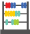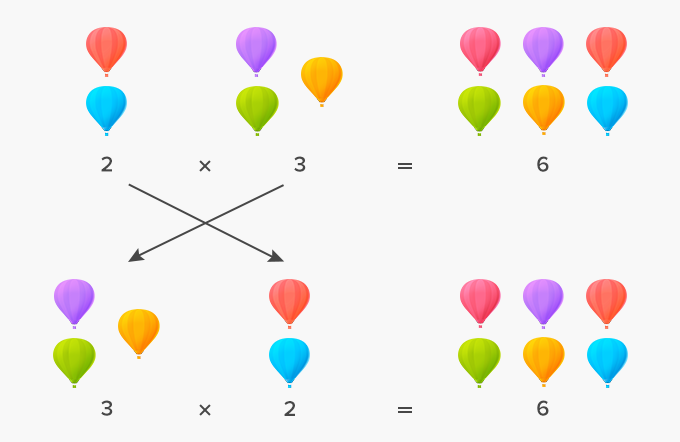# Commutative Property Of Multiplication - Definition with Examples

The Complete K-5 Math Learning Program Built for Your Child

• 40 Million Kids

Loved by kids and parent worldwide

• 50,000 Schools

Trusted by teachers across schools

• Comprehensive Curriculum

Aligned to Common Core

##Let's learn!

What is the commutative property of multiplication?
Commutative comes from the word “commute” which can be defined as to move around or travel.
According to the commutative property of multiplication, changing the order of the numbers we are multiplying, does not change the product

Here’s an example of how the product does NOT change, even if the order of the factors is changed.Here’s another example.

 7 x 8 x 11 x 2 = 11 x 7 x 2 x 8                          1232 = 1232

 Fun Facts This property is also called the order property of multiplication. Commutative property only applies to multiplication and addition. However, subtraction and division are not commutative.

##Let's sing!

When multiplying numbers two or more,
Change the order, but you will still score!
Don’t let multiplying in order, be the aim,
Because the product will just be the same!

##Let's do it!

Take your child out to a bakery shop. Ask them to calculate the approximate number of cookies in ten packs or the number of pastries in ten trays. Ask the children to multiply to find the total number of cookies or pastries. Ask them to check their calculations using the commutative property of multiplication.

##Related math vocabulary

Won Numerous Awards & Honors